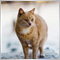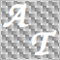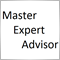• 概述
• 评论 (3)
• 评论

# Moving Average Envelopes tfmt4

Moving Average Envelopes use a percentage of a simple moving average to create an upper and lower envelope around the moving average. Using the middle moving average value, the chosen percentage from the input is multiplied by the MA value to add upper and lower envelopes. As the MA value is different among markets and currency pairs, you must manually adjust the chosen percentage for each chart. If you do not see the envelopes on a chart, change the percentage to a smaller value until you see the envelopes.

Typical use is to see when the price breaks out of the envelope as this may indicate the beginning of a trend.

You can change the moving average and the percentage of the moving average. The screenshot shows the percent input value of 1.25% entered as 1.25 on the EUR/USD chart. The inputs include:

• Periods: The number of bars to use in the simple moving average based on the close
• Percent: The percentage to multiply by the moving average value to create the envelopes8381
2018.12.16 05:03

Thanks for sharing21651
2016.08.31 18:52261
2014.07.06 02:22